# AIIMS Chemistry Mock Test - 10

## 60 Questions MCQ Test AIIMS Mock Tests & Previous Year Papers | AIIMS Chemistry Mock Test - 10

Description
Attempt AIIMS Chemistry Mock Test - 10 | 60 questions in 60 minutes | Mock test for NEET preparation | Free important questions MCQ to study AIIMS Mock Tests & Previous Year Papers for NEET Exam | Download free PDF with solutions
QUESTION: 1

### Which of the following is not formed when glycerol reacts with HI?

Solution: Because in options a & c the structure is stabilized by resonance, in option d there is only -I effect of I and in option b there is -I effect of I and -OH is present which makes that structure highly unstable .... So option b being highly unstable it can't be possible.
QUESTION: 2

Solution:
QUESTION: 3

### 'M' is the molecular mass of 'KMnO₄'.The equivalent mass of KMnO₄ when it is converted into K₂MnO₄ is

Solution:
QUESTION: 4

Among the following compounds the one that is polar and has the central atom with sp2 hybridisation is

Solution:
QUESTION: 5

Ethylbenzene with Br₂ in the presence of FeBr₃ gives

Solution: Bromine is ortho -para directing group . so, when ethyl benzene reacts with Ba2 in presence of FeBr3 it produces o- & p- bromoethylbenzene.
QUESTION: 6

The compound used as refrigerant is

Solution:
QUESTION: 7

Which of the following gas changes lime water in milky colour?

Solution:
QUESTION: 8

In a substitution reaction of C₆H₅Y, the major product is m-isomer, therefore the group Y can be

Solution:
QUESTION: 9

Which of the following is most reactive towards electrophilic substitution ?

Solution:
QUESTION: 10

The rate of reaction is doubled for 10º rise in temperature. The increase in the reaction rate as a result of temperature rise from 10º to 100º is

Solution:
QUESTION: 11

Variation of heat of reaction with temperature is known as

Solution:
QUESTION: 12

if the rate of the reaction is equal to the rate constant, the order of the reaction is

Solution:
QUESTION: 13

In the following question, a Statement of Assertion (A) is given followed by a corresponding Reason (R) just below it. Read the Statements carefully and mark the correct answer-
Assertion(A): Hard water is more suitable than soft water for laundary and dying.
Reason(R): Hard water can be used in steam boilers.

Solution:
QUESTION: 14

In the following question, a Statement of Assertion (A) is given followed by a corresponding Reason (R) just below it. Read the Statements carefully and mark the correct answer-
Assertion(A): Equal volume of two gases A and B diffuse through a porous pot in 20 and 10 seconds respectively.
Reason (R): Molar mass of A is 80 and B is 20.

Solution:
QUESTION: 15

In the following question, a Statement of Assertion (A) is given followed by a corresponding Reason (R) just below it. Read the Statements carefully and mark the correct answer-
Assertion(A): A universal indicator is useful in all acid base titrations.
Reason(R): Universal indicator has a limited pH range like any other indicator.

Solution:
QUESTION: 16

In the following question, a Statement of Assertion (A) is given followed by a corresponding Reason (R) just below it. Read the Statements carefully and mark the correct answer-
Assertion(A): BF3 is a weaker Lewis acid than BCl3.
Reason(R): The planar BF3 molecule is stabilised to a greater extent than BCl3 by B-X π bonding.

Solution:
QUESTION: 17

In the following question, a Statement of Assertion (A) is given followed by a corresponding Reason (R) just below it. Read the Statements carefully and mark the correct answer-
Assertion(A): Ammonolysis of alkyl halides ivolves the reaction between alkyl halides and alcoholic ammonia.
Reason(R): Reaction can be used to prepare only 2° amines.

Solution:
QUESTION: 18

In the following question, a Statement of Assertion (A) is given followed by a corresponding Reason (R) just below it. Read the Statements carefully and mark the correct answer-
Assertion(A): Lactice acid is optically active.
Reason(R): A symmetry in the organic compound causes optical activity.

Solution:
QUESTION: 19

In the following question, a Statement of Assertion (A) is given followed by a corresponding Reason (R) just below it. Read the Statements carefully and mark the correct answer-
Assertion(A): All F-S-F angle in SF4 is less than 180 degrees.
Reason(R): The lone pair-bond pair repulsion is weaker than bond pair-bond pair repulsion.

Solution:
QUESTION: 20

In the following question, a Statement of Assertion (A) is given followed by a corresponding Reason (R) just below it. Read the Statements carefully and mark the correct answer-
Assertion(A): About 50% of energy released during degradation of glucose appears as heat. The remainder is converted to chemical energy and stored in ATP.
Reason(R): ATP accumulate energy and store it for later use.

Solution:
QUESTION: 21

In the following question, a Statement of Assertion (A) is given followed by a corresponding Reason (R) just below it. Read the Statements carefully and mark the correct answer-
Assertion(A): Caro's acid has S atom in +6 oxidation state.
Reason(R): Caro's acid contains one peroxo O22- group.

Solution:
QUESTION: 22

In the following question, a Statement of Assertion (A) is given followed by a corresponding Reason (R) just below it. Read the Statements carefully and mark the correct answer-
Assertion(A): Concentration of H₃O⁺ ion and OH⁻ ion in pure water is same at 25ºC.
Reason(R): Pure water on self ionisation gives little more H₃O⁺ than OH⁻ ions at 25ºC.

Solution:
QUESTION: 23

In the following question, a Statement of Assertion (A) is given followed by a corresponding Reason (R) just below it. Read the Statements carefully and mark the correct answer-
Assertion(A): CO₂ follows PV = nRT equation at a low temperature and high pressure.
Reason(R): CO₂, may behave as an ideal gas when force of attraction among its molecules is negligible.

Solution:
QUESTION: 24

In the following question, a Statement of Assertion (A) is given followed by a corresponding Reason (R) just below it. Read the Statements carefully and mark the correct answer-
Assertion(A): SiO2 is solid while CO2 is gas at room temperature.
Reason(R): Si-O bonds are more stronger than C-O bonds.

Solution:
QUESTION: 25

In the following question, a Statement of Assertion (A) is given followed by a corresponding Reason (R) just below it. Read the Statements carefully and mark the correct answer-
Assertion(A): When CoCl3.5NH3 is dissolved in water and treated with excess AgNO3 solution , it gives only two moles of AgCl.
Reason(R): Third chloro is hydrogen bonded to NH3.

Solution:
QUESTION: 26

In the following question, a Statement of Assertion (A) is given followed by a corresponding Reason (R) just below it. Read the Statements carefully and mark the correct answer-
Assertion(A): A mixture of the solutions of a weak acid and its sodium salt acts as a good buffer.
Reason(R): The ratio of the sale to acid in the mixture does not change substantially when small amounts of acids or alkalies are added to the buffer.

Solution:
QUESTION: 27

In the following question, a Statement of Assertion (A) is given followed by a corresponding Reason (R) just below it. Read the Statements carefully and mark the correct answer-
Assertion(A): Each carbon in ethylene molecule is sp2 hydridised.
Reason(R): The H-C-H bond angle in ethylene molecule is 120º.

Solution:
QUESTION: 28

In the following question, a Statement of Assertion (A) is given followed by a corresponding Reason (R) just below it. Read the Statements carefully and mark the correct answer-
Assertion(A): p-dimethylamino-benzaldehyde does not undergo benzoin condensation.
Reason(R): The aldehydic (-CHO) group is meta directing .

Solution:
QUESTION: 29

In the following question, a Statement of Assertion (A) is given followed by a corresponding Reason (R) just below it. Read the Statements carefully and mark the correct answer-
Assertion(A): Li and Mg form nitride but not Na when heated in air.
Reason(R): Na only forms oxide due to its high affinity for oxygen.

Solution:
QUESTION: 30

In the following question, a Statement of Assertion (A) is given followed by a corresponding Reason (R) just below it. Read the Statements carefully and mark the correct answer-
Assertion(A): Molar conductance increases with dilution for an electrolyte solution while its specific conductance decreases.
Reason(R): The number of ions in one litre of electrolyte increases with dilution.

Solution:
QUESTION: 31

In the following question, a Statement of Assertion (A) is given followed by a corresponding Reason (R) just below it. Read the Statements carefully and mark the correct answer-
Assertion(A): Al(OH)3 is amphoteric in nature.
Reason(R): Al-O and O-H bonds can be broken with equal ease in Al(OH)3.

Solution:
QUESTION: 32

In the following question, a Statement of Assertion (A) is given followed by a corresponding Reason (R) just below it. Read the Statements carefully and mark the correct answer-
Assertion(A): In CaCl crystal, the co-ordination number of Cs+ ion is 8.
Reason(R): Ci-ion in CsCl adopt bcc type of packing.

Solution:
QUESTION: 33

The quanitity of electricity required to librate 112 cm3 of hydrogen at STP from acidified water is

Solution:

2H+ + 2e- → H2
2e- → 2F
H2 → 22.4 L at STP
To liberate 112cm3 at STP electron required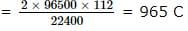QUESTION: 34

Molar ionic conductivities of a bivalent electrolyte are 57 and 73. The molar conductivity of the solution will be

Solution:
QUESTION: 35

According to kinetic theory of gases, a gas molecule travels between two successive collisions

Solution:
QUESTION: 36

Which of the following imparts violet colouration to the non-luminous flame of Bunsen burner?

Solution:
QUESTION: 37

An aqueous solution of sodium carbonate is alkaline because sodium carbonate is a salt of

Solution:
QUESTION: 38

Which of the following oxidation state is/are exhibited by alkali metals in their compounds?

Solution:
QUESTION: 39

Which one of the following species is not a pseudohalide?

Solution:
QUESTION: 40

Which of the following compound of an alkaline earth metal is extremely hard?

Solution:
QUESTION: 41

Which among the following has the largest dipolemoment

Solution:
QUESTION: 42

Which one of the following is acid salt?

Solution:
QUESTION: 43

To a solution containing equimolar mixture of sodium acetate and acetic acid, some more amount of sodium acetate solution is added. The pH of mixture solution

Solution:
QUESTION: 44

Assuming complete ionization, the pH of 0.1 M, HCl is 1. The molarity of H₂SO₄ with the same pH is

Solution:
QUESTION: 45

A mixture of a weak acid (say acetic acid )and its salt with a strong base (say sodium acetate )is a buffer solution.Which other pair of substances from the following may have a similar property ?

Solution:
QUESTION: 46

Reduction of > C═O to CH₂ can be carried out with

Solution:
QUESTION: 47

Which of the following is a mixed ketone?

Solution:
QUESTION: 48

Which produces the best electrophile?

Solution:
QUESTION: 49

Select the alkene(s) in which addition of HX is strictly regiospecific: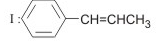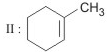IV: CH3CH═CH2

Solution:
QUESTION: 50

Most stable alkene is :

Solution:
QUESTION: 51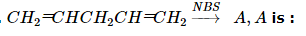Solution:
QUESTION: 52

p-type silicon is formed by adding a minute amount of an element with

Solution:
QUESTION: 53

To obtain pure silicon, SiH₄ is heated at

Solution:
QUESTION: 54

A face-centred cubic element has a edge length of 400 pm. What is its density? At. weight of element= 60)

Solution:
QUESTION: 55

The volume of water to be added to 100 cm3 of 0.5 NH₂SO₄ to get dicinormal concentration is

Solution:
QUESTION: 56

The relative lowering of vapour pressure is equal to the mole fraction of the solute. This law is called

Solution:
QUESTION: 57

The weight in grams of KCl(mol.wt. = 74.5) in 100ml of a 0.1M KCl solution is

Solution:
QUESTION: 58

Which of the following would not have a trans isomer?

Solution:
QUESTION: 59

The number of moles of oxygen in one litre of air containing 21% oxygen by volume, in standard conditions, is

Solution:

Amount of O 2 in 1 litre air = 21 % = 0.21 L .

∵ 22.4 L . of O 2 = 1 mol
∴ 0.21 L of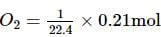= 0.0093 mol

QUESTION: 60

Identify the statement which is not correct regarding CuSO₄.

Solution:Use Code STAYHOME200 and get INR 200 additional OFF Use Coupon Code

### How to Prepare for NEET

Read our guide to prepare for NEET which is created by Toppers & the best Teachers